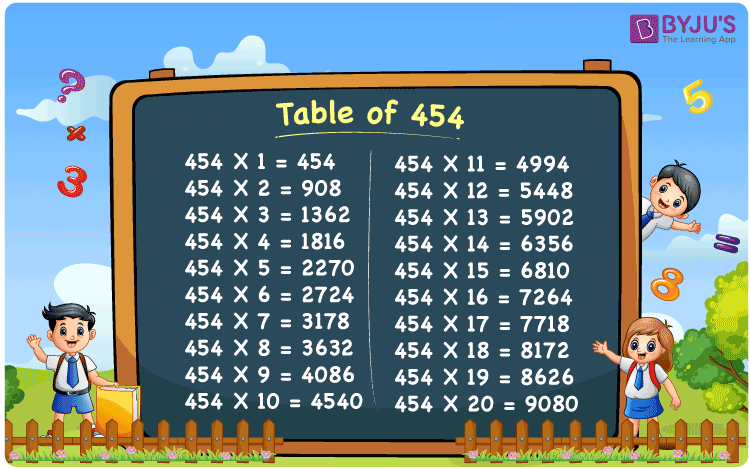Checkout JEE MAINS 2022 Question Paper Analysis : Checkout JEE MAINS 2022 Question Paper Analysis :

# Table of 454

The Table of 454 is presented here in table grid format to assist students in quickly checking the values of multiples of 454. Solved examples are also available to assist with questions about the table of 454. Students can use a downloadable PDF of the multiplication table of 454 to memorise multiples of 454, and retain it for future reference.

## Table of 454 Chart## What is the 454 Times Table?

The 454 times table is the list of all multiples of 454. Any natural number multiplied by 454 is the multiple of 454. The multiplication table of 454 can be represented in both multiplication and addition form. The below table lists the multiples of 454 in both multiplication and addition form.

 454×1 = 454 454 454×2 = 908 454 + 454 = 908 454×3 = 1362 454 + 454 + 454 = 1362 454×4 = 1816 454 + 454 + 454 + 454 = 1816 454×5 = 2270 454 + 454 + 454 + 454 + 454 = 2270 454×6 = 2724 454 + 454 + 454 + 454 + 454 + 454 = 2724 454×7 = 3178 454 + 454 + 454 + 454 + 454 + 454 + 454 = 3178 454×8 = 3632 454 + 454 + 454 + 454 + 454 + 454 + 454 + 454 = 3632 454×9 = 4086 454 + 454 + 454 + 454 + 454 + 454 + 454 + 454 + 454 = 4086 454×10 = 4540 454 + 454 + 454 + 454 + 454 + 454 + 454 + 454 + 454 + 454 = 4540

## Multiplication Table of 454

The multiplication table of 454 is given below in tabular form:

 454 × 1 = 454 454 × 2 = 908 454 × 3 = 1362 454 × 4 = 1816 454 × 5 = 2270 454 × 6 = 2724 454 × 7 = 3178 454 × 8 = 3632 454 × 9 = 4086 454 × 10 = 4540 454 × 11 = 4994 454 × 12 = 5448 454 × 13 = 5902 454 × 14 = 6356 454 × 15 = 6810 454 × 16 = 7264 454 × 17 = 7718 454 × 18 = 8172 454 × 19 = 8626 454 × 20 = 9080

## Solved Example on Table of 454

Q. What is the difference between the 18th multiple and the 20th multiple of 454?

Solution:

Using the multiplication table of 454

The 18th multiple of 454 = 8172

The 20th multiple of 454 = 9080

The difference between the 18th multiple and 20th multiple = 9080 – 8172 = 908

## Frequently Asked Questions on the Table of 454

### What is the table of 454?

The table of 454 is the list of all multiples of 454 starting from 454 × 1 = 454, 454 × 2 = 908, 454 × 3 = 1362, and so on.

### What is 5 times 454?

5 times 454 is 2270.

### Calculate the value of 454 times 10 minus 40.

454 times 10 minus 40 equals 4500.